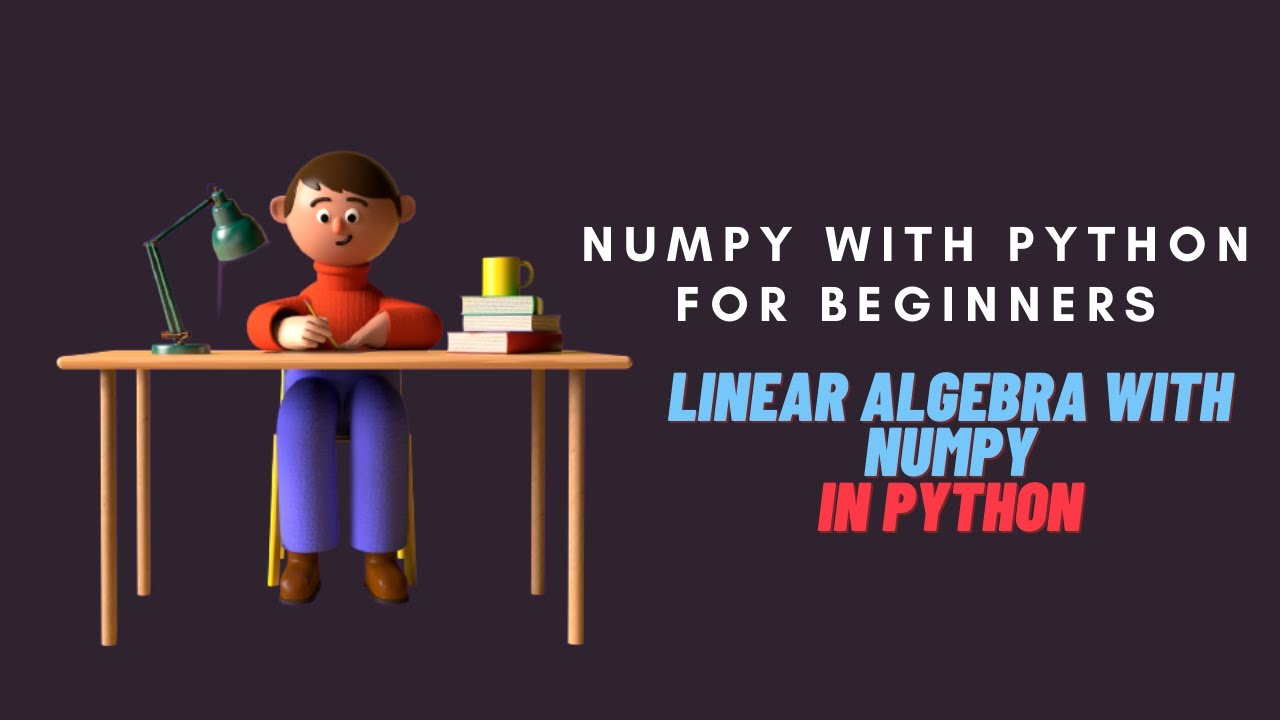1625595120

# Numpy with Python Tutorial for Beginners | Linear Algebra with Numpy in Python

In this ‘Python Tutorial for Beginners’, we will learn
What is Numpy Library in Python?
What is Scipy Library in Python?
How we can use Numpy Library with Python?
How we can use Scipy Library with Python?
What is Reverse List Indexing and Slicing?

How we can use Numpy Library for Linear Algebra with Python?
How we can use Scipy Library for Linear Algebra with Python?

This video is a Python Tutorial to learn Python programming with examples

In this video we will see :

• NumPy is a Python library used for working with arrays. It also has functions for working in domain of linear algebra, fourier transform, and matrices. NumPy was created in 2005 by Travis Oliphant. It is an open source project and you can use it freely. NumPy stands for Numerical Python.

• The SciPy library is one of the core packages that make up the SciPy stack. It provides many user-friendly and efficient numerical routines, such as routines for numerical integration, interpolation, optimization, linear algebra, and statistics. … SciPy is a community-driven project.

Complete Blog on ‘Python for Absolute Beginners’ -
https://seeve.medium.com/python-for-absolute-beginners-d6d967b28552

#python